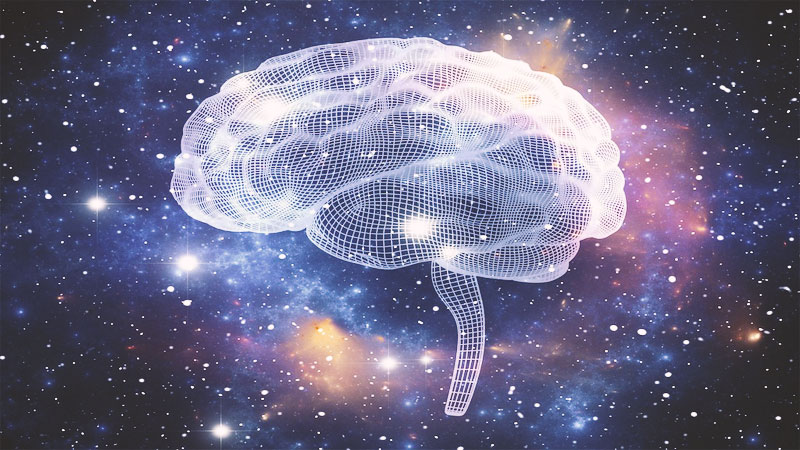# Universe: An Interconnected Neural Network?

A new way of thinking is emerging about how different areas of physics could be connected to create a model that unites traditional scientific thinking with new ideas in quantum physics.For years, the most brilliant physicists have tried to unify Classical Physics and Quantum Physics into a “Theory of Everything” without success so far.

Classical physics dates back to the time of Isaac Newton and is based on physical and mechanical equations in which everything works like clockwork, in a predictable and known way.

Quantum physics, on the other hand, looks at microscopic and subatomic scales and how they interact at the levels of particles, waves, and force fields, where the fundamental laws at this quantum level are opposite to their behavior at the classical level. Instead of certainty you have uncertainty, instead of predictability you have probability.

So how do we connect these different views with the so-called “Theory of Everything”?

A recent paper by Vitaly Vanchurin, professor of physics at the University of Minnesota, argues that rather than simply looking to bridge the relativistic and quantum worlds, perhaps a third phenomenon deserves to be incorporated as well: that of observers.

In his article, Vanchurin considers the possibility that a proposed “microscopic neural network” could serve as the fundamental framework from which all other phenomena in nature – quantum, classical and macroscopic observers – emerge.

The article builds on a previous article, “Towards a Theory of Machine Learning,” in which Vanchurin employed statistical mechanics to examine neural networks. From this work, Vanchurin first became aware of some of the corollaries that appear to exist between neural networks and the dynamics of quantum physics.

“We discussed the possibility that the entire universe at its most fundamental level is a neural network. We have identified two different types of dynamic degrees of freedom: “trainable” variables (eg, polarization vector or weight matrix) and “hidden” variables (eg, state vector of neurons).

We first consider the evolution of the probabilities of the trainable variables to argue that close to equilibrium their dynamics are well approximated by Madelung’s equations (with free energy representing the phase) and further from equilibrium by the Hamilton-Jacobi equations (with free energy representing Hamilton’s main function).

This shows that trainable variables can indeed exhibit classical and quantum behavior with the state vector of neurons representing the hidden variables. If the subsystems are interacting minimally, with interactions described by a metric tensor, then the emergent spacetime becomes curved.

We argue that the entropy production in such a system is a local function of the metric tensor which must be determined by the symmetries of the Onsager tensor. It turns out that a very simple and highly symmetric Onsager tensor leads to the entropy production described by Einstein and Hilbert. This shows that the learning dynamics of a neural network can indeed exhibit approximate behaviors described by both quantum mechanics and general relativity. We also discussed the possibility that the two descriptions are dual holographic forms of each other.”